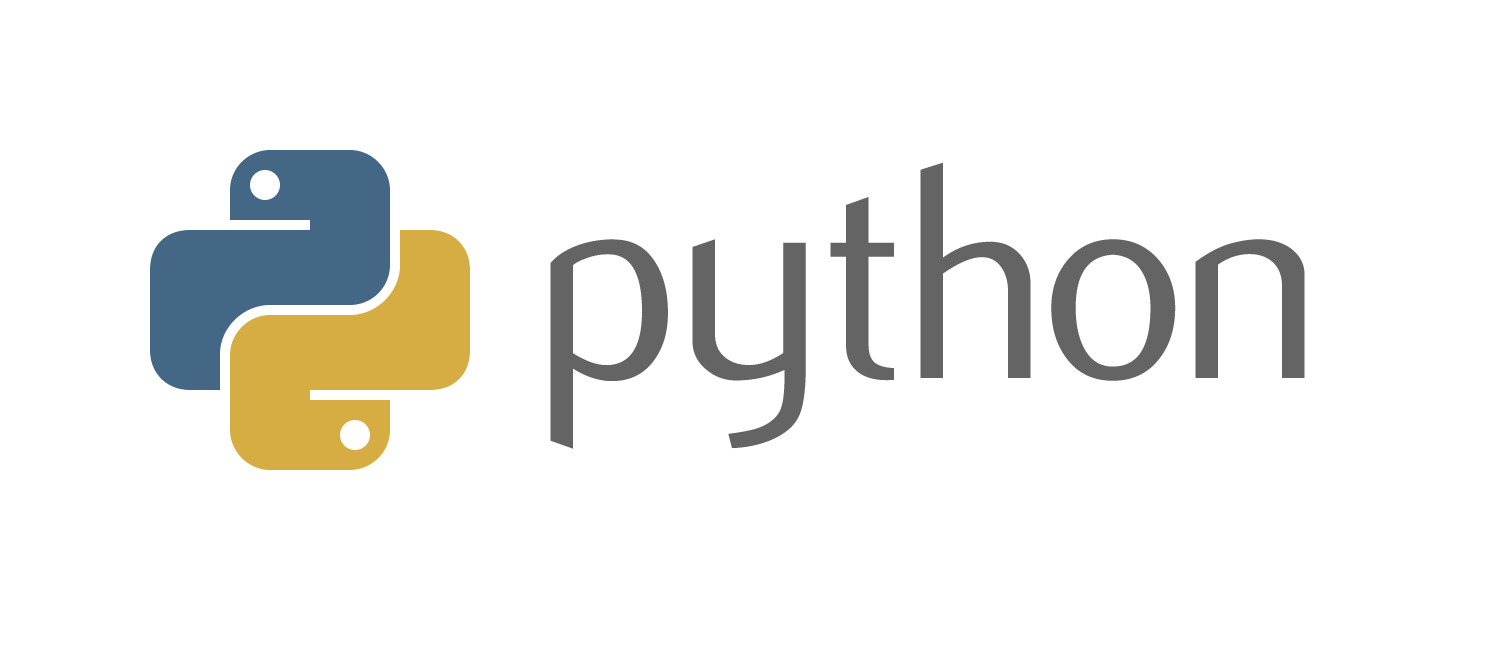# Basic Tutorials Part 5

Basic Tutorials Part 5## Python Basics

In this post we discuss the Python Programming basics.

An overview of why we want to use the langauge has been given in Part 0 of this series.

This post shall serve as an introductory-crash course to Python.

Here is the accompanying Notebook, the code portions discussed here and in the Notebook will be with bits and pieces left for the reader to figure out. We expect a more active participation in learning. Do leave a comment below if you feel anything is missing or have any doubts.

Python Shell

``````python
``````

In a terminal fires up a Python shell.

We suggest you use Jupyter notebooks (Details were discussed in the early posts)

• Printing
``````print(varName)
print('hello world')
``````
• For loops
``````
for i in range(4):
for j in range(4):
if j > i:
break
print((i, j))
``````

Notice the indents. Python uses indentation instead of using braces to mark the bodies.

• Tuples

Tuples can be created by () braces

``````tup = 4, 5, 6
``````
• List

Lists are created by enclosing within [] braces

``````a_list = [2, 3, 7, None]
tup = ('foo', 'bar', 'baz')
b_list = list(tup)
b_list
b_list = 'peekaboo'
b_list
``````

Lists can be roughly linked to deques in their functionality

• Dictionary

Dictionaries store values in the form of Key Value Pairs

``````empty_dict = {}
d1 = {'a' : 'some value', 'b' : [1, 2, 3, 4]}
d1
``````
• List Comprehensions:

List comprehensions are a neat trick to collapse several lines of codes into one

``````strings = ['a', 'as', 'bat', 'car', 'dove', 'python']
[x.upper() for x in strings if len(x) > 2]
``````
``````Leave us a comment below if there is anything you want to discuss.

Subscribe to my weekly-ish [Newsletter](https://tinyletter.com/sanyambhutani/) for a set of curated Deep Learning reads

``````